Here is the final tutorial of this long series. We’re going to see how to apply a texture to a mesh by using mapping coordinates exported from Blender. If you’ve managed to understand the previous tutorials, it will just be some piece of cake to apply some textures. The main concept is once again to interpolate some data between each vertex. In the second part of this tutorial, we’ll see how to boost the performance of our rendering algorithm. For that, we’re going to only display visible faces by using a back-face culling approach. But to go even further, we will use our last secret weapon: the GPU.

You will then understand why OpenGL/WebGL and DirectX technologies are so important to make realtime 3D games. They help to use the GPU instead of the CPU to render our 3D objects. To really see the differences, we will load the exact same JSON file inside a WebGL 3D engine named Babylon.JS. The rendering will be far better and the FPS will be without any comparison, especially on low-end devices!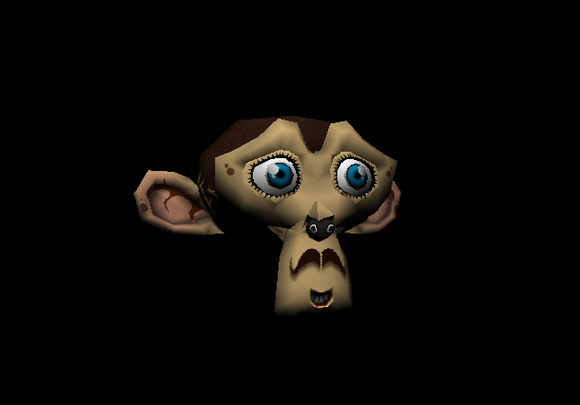At the end of this tutorial, you will have this final rendering inside our CPU-based 3D software engine:

As a reminder, this tutorial is part of the following series:

### Texture mapping Concept

Let’s start by the Wikipedia definition: Texture mapping : “A texture map is applied (mapped) to the surface of a shape or polygon. This process is akin to applying patterned paper to a plain white box. Every vertex in a polygon is assigned a texture coordinate (which in the 2d case is also known as a UV coordinate) either via explicit assignment or by procedural definition. Image sampling locations are then interpolated across the face of a polygon to produce a visual result that seems to have more richness than could otherwise be achieved with a limited number of polygons.

Let’s now try to understand what this means exactly.

The first time I’ve tried to imagine how we could apply a texture to a 3D mesh was by first thinking about a cube, the first mesh we’ve drawn in this series. I was then thinking about taking an image acting as our texture and map it to each cube’s faces. This could work well in such a simple case. But the first problem will be: what if I’d like to apply a different image/texture on each cube’s faces? A first idea could be to take 6 different images for the 6 sides of your cube. To be even more precise, take 6 images, split them into 2 triangles that will be mapped to the 12 triangles of a cube.

But there is a simpler more elegant approach you’ve probably already played with when you were a child. This image will help you: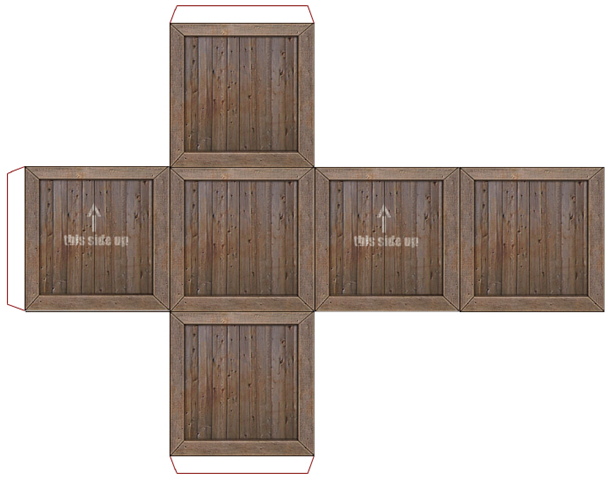The very same approach works perfectly well with 3D engine. Imagine this image as the texture that will be applied to our cube. View it as 2 dimensional array of color’s bytes. We will be able to affect some 2D coordinates moving into this array to each cube’s vertex in order to obtain something like that: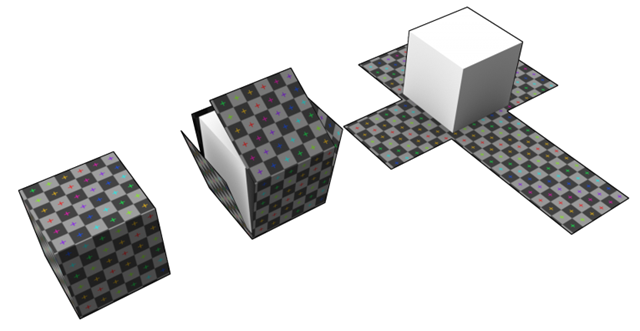Image taken from this forum thread: Texturing a cube in Blender, and going to pull my hair out

These 2D texture coordinates are known as UV coordinates.

Note: I’ve asked to a 3D guru what were the reasons to name them U & V? The answer was amazingly obvious: “Well, it’s because it’s just before X, Y, Z.”. I was expecting a more complex answer! ;)

You’re now probably asking to yourself how to handle advanced complex meshes like Suzanne, our beautiful monkey’s head, aren’t you?

For this kind of mesh, we’re also going to use a single 2D image that will be mapped in 3D. To build the corresponding texture, we need a planned 2D view of your mesh. This operation is known as an unwrap operation. If you’re a poor developer like myself, trust me, you’ll need a brilliant 3D designer like my friend Michel Rousseau to help you in this phase! And this is exactly what I’ve done: asking for help. :)

Using the Suzanne model as an example, after the unwrap operation, the designer will obtain this kind of result: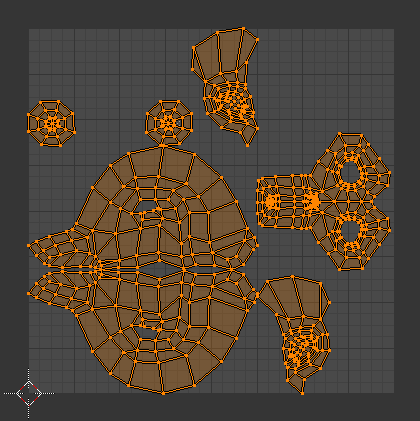The designer will then paint this planned 2D view and the result will be the texture ready to be used by our engine. In our case, Michel Rousseau has done this job for us and here is his own version of Suzanne: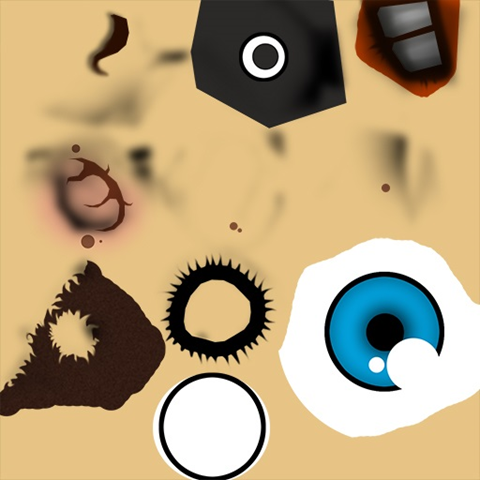I know this result may looks weird the first time you try to understand texture mapping. But you should already see something that looks like an eye in the bottom right of the texture. This part will be mapped in 3D to both Suzanne’s eyes using a simple symmetrical operation to differentiate both eyes.

You now known the basics of texture’s mapping. To definitely understand how it works, please read these additional resources I’ve found for you on the web:

Tutorial 16 – Basic Texture Mapping, read the first part that will help to understand how to map the UV coordinates (living between 0 and 1) to the triangles of our meshes
Blender 2.6 Manual – UV Mapping a Mesh, that describes the various mapping types
Tutorial 5 – Texture mapping, read the first part that will definitely help you to at least know how to map a cube. :)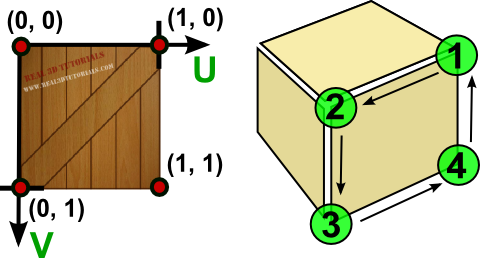### Code

We’re now ready to dig into the code. There are several tasks to be done :

1 – Create a Texture class that will load the image acting as the texture and return the color associated to the U & V coordinates interpolated per pixel
2 – Add/Pass the Texture information in the complete rendering flow
3 – Parse the JSON file exported by the Babylon Blender’s Add-on to load the UV coordinates

### The Texture logic

In HTML5 with TypeScript/JavaScript, we’re going of course to loading the texture by dynamically creating a canvas element and getting it’s associated image data to obtain our color bytes array.

With C#/XAML, we’re going to create a WriteableBitmap, set its source with the image we will load and get its PixelBuffer property to obtain our color bytes array.

```public class Texture
{
private byte[] internalBuffer;
private int width;
private int height;
// Working with a fix sized texture (512x512, 1024x1024, etc.).
public Texture(string filename, int width, int height)
{
this.width = width;
this.height = height;
}
{
var file = await Windows.ApplicationModel.Package.Current.InstalledLocation.GetFileAsync(filename);
using (var stream = await file.OpenReadAsync())
{
var bmp = new WriteableBitmap(width, height);
bmp.SetSource(stream);
internalBuffer = bmp.PixelBuffer.ToArray();
}
}
// Takes the U & V coordinates exported by Blender
// and return the corresponding pixel color in the texture
public Color4 Map(float tu, float tv)
{
// Image is not loaded yet
if (internalBuffer == null)
{
return Color4.White;
}
// using a % operator to cycle/repeat the texture if needed
int u = Math.Abs((int) (tu*width) % width);
int v = Math.Abs((int) (tv*height) % height);
int pos = (u + v * width) * 4;
byte b = internalBuffer[pos];
byte g = internalBuffer[pos + 1];
byte r = internalBuffer[pos + 2];
byte a = internalBuffer[pos + 3];
return new Color4(r / 255.0f, g / 255.0f, b / 255.0f, a / 255.0f);
}
```

```export class Texture {
width: number;
height: number;
internalBuffer: ImageData;
// Working with a fix sized texture (512x512, 1024x1024, etc.).
constructor(filename: string, width: number, height: number) {
this.width = width;
this.height = height;
}
var imageTexture = new Image();
imageTexture.height = this.height;
imageTexture.width = this.width;
var internalCanvas: HTMLCanvasElement = document.createElement("canvas");
internalCanvas.width = this.width;
internalCanvas.height = this.height;
var internalContext: CanvasRenderingContext2D = internalCanvas.getContext("2d");
internalContext.drawImage(imageTexture, 0, 0);
this.internalBuffer = internalContext.getImageData(0, 0, this.width, this.height);
};
imageTexture.src = filename;
}
// Takes the U & V coordinates exported by Blender
// and return the corresponding pixel color in the texture
public map(tu: number, tv: number): BABYLON.Color4 {
if (this.internalBuffer) {
// using a % operator to cycle/repeat the texture if needed
var u = Math.abs(((tu * this.width) % this.width)) >> 0;
var v = Math.abs(((tv * this.height) % this.height)) >> 0;
var pos = (u + v * this.width) * 4;
var r = this.internalBuffer.data[pos];
var g = this.internalBuffer.data[pos + 1];
var b = this.internalBuffer.data[pos + 2];
var a = this.internalBuffer.data[pos + 3];
return new BABYLON.Color4(r / 255.0, g / 255.0, b / 255.0, a / 255.0);
}
// Image is not loaded yet
else {
return new BABYLON.Color4(1, 1, 1, 1);
}
}
```

```var Texture = (function () {
// Working with a fix sized texture (512x512, 1024x1024, etc.).
function Texture(filename, width, height) {
this.width = width;
this.height = height;
}
var _this = this;
var imageTexture = new Image();
imageTexture.height = this.height;
imageTexture.width = this.width;
var internalCanvas = document.createElement("canvas");
internalCanvas.width = _this.width;
internalCanvas.height = _this.height;
var internalContext = internalCanvas.getContext("2d");
internalContext.drawImage(imageTexture, 0, 0);
_this.internalBuffer = internalContext.getImageData(0, 0, _this.width, _this.height);
};
imageTexture.src = filename;
};
// Takes the U & V coordinates exported by Blender
// and return the corresponding pixel color in the texture
Texture.prototype.map = function (tu, tv) {
if (this.internalBuffer) {
// using a % operator to cycle/repeat the texture if needed
var u = Math.abs(((tu * this.width) % this.width)) >> 0;
var v = Math.abs(((tv * this.height) % this.height)) >> 0;
var pos = (u + v * this.width) * 4;
var r = this.internalBuffer.data[pos];
var g = this.internalBuffer.data[pos + 1];
var b = this.internalBuffer.data[pos + 2];
var a = this.internalBuffer.data[pos + 3];
return new BABYLON.Color4(r / 255.0, g / 255.0, b / 255.0, a / 255.0);
}
// Image is not loaded yet
else {
return new BABYLON.Color4(1, 1, 1, 1);
}
};
return Texture;
)();
oftEngine.Texture = Texture;```

### Pass the Texture information in the flow

I won’t dig into every detail as you’ve got the complete source to download a bit below. Let’s rather review globally what you need to do:

– add a Texture property to the Mesh class and a Vector2 property named TextureCoordinates to the Vertex structure

– update ScanLineData to embed 8 more floats/numbers : the UV coordinates per vertex (ua, ub, uc, ud & va, vb, vc, vd).

– update the Project method/function to return a new Vertex with the TextureCoordinates passed as-is (pass through)

– pass a Texture object as the last parameter to the ProcessScanLine, DrawTriangle methods/functions

– Fill the new ScanLineData structure in drawTriangle with the appropriate UV coordinates

Interpolate the UV in ProcessScanLine on Y to have SU/SV & EU/EV (start U/start V/End U/End V) then interpolate U, V on X, find the corresponding color with it in the texture. This color texture will be mixed with the native object’s color (always white in our tutorials case) and the light quantity measured with the NDotL operation with the normal.

Note: our Project method could be seen as what we name a “Vertex Shader” in a 3D hardware engine and our ProcessScanLine could be seen as a “Pixel Shader”.

I’m sharing in this article only the new ProcessScanLine method which is really the main part to be updated:

```void ProcessScanLine(ScanLineData data, Vertex va, Vertex vb, Vertex vc, Vertex vd, Color4 color, Texture texture)
Vector3 pa = va.Coordinates;
Vector3 pb = vb.Coordinates;
Vector3 pc = vc.Coordinates;
Vector3 pd = vd.Coordinates;
// Thanks to current Y, we can compute the gradient to compute others values like
// the starting X (sx) and ending X (ex) to draw between
// if pa.Y == pb.Y or pc.Y == pd.Y, gradient is forced to 1
var gradient1 = pa.Y != pb.Y ? (data.currentY - pa.Y) / (pb.Y - pa.Y) : 1;
var gradient2 = pc.Y != pd.Y ? (data.currentY - pc.Y) / (pd.Y - pc.Y) : 1;
int sx = (int)Interpolate(pa.X, pb.X, gradient1);
int ex = (int)Interpolate(pc.X, pd.X, gradient2);
// starting Z & ending Z
float z1 = Interpolate(pa.Z, pb.Z, gradient1);
float z2 = Interpolate(pc.Z, pd.Z, gradient2);
// Interpolating normals on Y
var snl = Interpolate(data.ndotla, data.ndotlb, gradient1);
var enl = Interpolate(data.ndotlc, data.ndotld, gradient2);
// Interpolating texture coordinates on Y
var su = Interpolate(data.ua, data.ub, gradient1);
var eu = Interpolate(data.uc, data.ud, gradient2);
var sv = Interpolate(data.va, data.vb, gradient1);
var ev = Interpolate(data.vc, data.vd, gradient2);
// drawing a line from left (sx) to right (ex)
for (var x = sx; x < ex; x++)
{
float gradient = (x - sx) / (float)(ex - sx);
// Interpolating Z, normal and texture coordinates on X
var z = Interpolate(z1, z2, gradient);
var ndotl = Interpolate(snl, enl, gradient);
var u = Interpolate(su, eu, gradient);
var v = Interpolate(sv, ev, gradient);
Color4 textureColor;
if (texture != null)
textureColor = texture.Map(u, v);
else
textureColor = new Color4(1, 1, 1, 1);
// changing the native color value using the cosine of the angle
// between the light vector and the normal vector
// and the texture color
DrawPoint(new Vector3(x, data.currentY, z), color * ndotl * textureColor);
}
```

```public processScanLine(data: ScanLineData, va: Vertex, vb: Vertex, vc: Vertex, vd: Vertex, color: BABYLON.Color4, texture?: Texture): void {
var pa = va.Coordinates;
var pb = vb.Coordinates;
var pc = vc.Coordinates;
var pd = vd.Coordinates;
// Thanks to current Y, we can compute the gradient to compute others values like
// the starting X (sx) and ending X (ex) to draw between
// if pa.Y == pb.Y or pc.Y == pd.Y, gradient is forced to 1
var gradient1 = pa.y != pb.y ? (data.currentY - pa.y) / (pb.y - pa.y) : 1;
var gradient2 = pc.y != pd.y ? (data.currentY - pc.y) / (pd.y - pc.y) : 1;
var sx = this.interpolate(pa.x, pb.x, gradient1) >> 0;
var ex = this.interpolate(pc.x, pd.x, gradient2) >> 0;
// starting Z & ending Z
var z1: number = this.interpolate(pa.z, pb.z, gradient1);
var z2: number = this.interpolate(pc.z, pd.z, gradient2);
// Interpolating normals on Y
var snl = this.interpolate(data.ndotla, data.ndotlb, gradient1);
var enl = this.interpolate(data.ndotlc, data.ndotld, gradient2);
// Interpolating texture coordinates on Y
var su = this.interpolate(data.ua, data.ub, gradient1);
var eu = this.interpolate(data.uc, data.ud, gradient2);
var sv = this.interpolate(data.va, data.vb, gradient1);
var ev = this.interpolate(data.vc, data.vd, gradient2);
// drawing a line from left (sx) to right (ex)
for (var x = sx; x < ex; x++) {
var gradient: number = (x - sx) / (ex - sx);
// Interpolating Z, normal and texture coordinates on X
var z = this.interpolate(z1, z2, gradient);
var ndotl = this.interpolate(snl, enl, gradient);
var u = this.interpolate(su, eu, gradient);
var v = this.interpolate(sv, ev, gradient);
var textureColor;
if (texture)
textureColor = texture.map(u, v);
else
textureColor = new BABYLON.Color4(1, 1, 1, 1);
// changing the native color value using the cosine of the angle
// between the light vector and the normal vector
// and the texture color
this.drawPoint(new BABYLON.Vector3(x, data.currentY, z),                        new BABYLON.Color4(color.r * ndotl * textureColor.r,                                           color.g * ndotl * textureColor.g,                                           color.b * ndotl * textureColor.b, 1));
}
```

```Device.prototype.processScanLine = function (data, va, vb, vc, vd, color, texture) {
var pa = va.Coordinates;
var pb = vb.Coordinates;
var pc = vc.Coordinates;
var pd = vd.Coordinates;
// Thanks to current Y, we can compute the gradient to compute others values like
// the starting X (sx) and ending X (ex) to draw between
// if pa.Y == pb.Y or pc.Y == pd.Y, gradient is forced to 1
var gradient1 = pa.y != pb.y ? (data.currentY - pa.y) / (pb.y - pa.y) : 1;
var gradient2 = pc.y != pd.y ? (data.currentY - pc.y) / (pd.y - pc.y) : 1;
var sx = this.interpolate(pa.x, pb.x, gradient1) >> 0;
var ex = this.interpolate(pc.x, pd.x, gradient2) >> 0;
// starting Z & ending Z
var z1 = this.interpolate(pa.z, pb.z, gradient1);
var z2 = this.interpolate(pc.z, pd.z, gradient2);
// Interpolating normals on Y
var snl = this.interpolate(data.ndotla, data.ndotlb, gradient1);
var enl = this.interpolate(data.ndotlc, data.ndotld, gradient2);
// Interpolating texture coordinates on Y
var su = this.interpolate(data.ua, data.ub, gradient1);
var eu = this.interpolate(data.uc, data.ud, gradient2);
var sv = this.interpolate(data.va, data.vb, gradient1);
var ev = this.interpolate(data.vc, data.vd, gradient2);
// drawing a line from left (sx) to right (ex)
for (var x = sx; x < ex; x++) {
var gradient = (x - sx) / (ex - sx);
// Interpolating Z, normal and texture coordinates on X
var z = this.interpolate(z1, z2, gradient);
var ndotl = this.interpolate(snl, enl, gradient);
var u = this.interpolate(su, eu, gradient);
var v = this.interpolate(sv, ev, gradient);
var textureColor;
if (texture)
textureColor = texture.map(u, v);
else
textureColor = new BABYLON.Color4(1, 1, 1, 1);
// changing the native color value using the cosine of the angle
// between the light vector and the normal vector
// and the texture color
this.drawPoint(new BABYLON.Vector3(x, data.currentY, z),                        new BABYLON.Color4(color.r * ndotl * textureColor.r,                                           color.g * ndotl * textureColor.g,                                           color.b * ndotl * textureColor.b, 1));
}
;```

If you’ve followed all the previous tutorials to build your own version, please download the code of my solutions to review other slight modifications to put in your own project.

To be able to have the nice rendering you’ve seen at the top of this article, you need to load a new version of Suzanne modified by Michel Rousseau and exported from Blender with the UV coordinates. For that, please download those 2 files:

– Suzanne Blender model with UV coordinates set: http://david.blob.core.windows.net/softengine3d/part6/monkey.babylon

– the 512×512 texture image to load with: http://david.blob.core.windows.net/softengine3d/part6/Suzanne.jpg

The Babylon.JSON format file of David Catuhe is containing a lot of details we won’t cover in this series. For instance, something that could be fun for you to play with is the material. Indeed, the designer can assign a particular material to a mesh. In our case, we’re only going to handle a diffuse texture. If you want to implement more of them, have a look to David Catuhe’s article as a base: Babylon.js: Unleash the StandardMaterial for your babylon.js game

Again, I’m sharing with you only the main part to change: the method/function loading & parsing the JSON file.

```// Loading the JSON file in an asynchronous manner
var meshes = new List<Mesh>();
var materials = new Dictionary<String,Material>();
var file = await Windows.ApplicationModel.Package.Current.InstalledLocation.GetFileAsync(fileName);
dynamic jsonObject = Newtonsoft.Json.JsonConvert.DeserializeObject(data);
for (var materialIndex = 0; materialIndex < jsonObject.materials.Count; materialIndex++)
{
var material = new Material();
material.Name = jsonObject.materials[materialIndex].name.Value;
material.ID = jsonObject.materials[materialIndex].id.Value;
if (jsonObject.materials[materialIndex].diffuseTexture != null)
material.DiffuseTextureName = jsonObject.materials[materialIndex].diffuseTexture.name.Value;
}
for (var meshIndex = 0; meshIndex < jsonObject.meshes.Count; meshIndex++)
{
var verticesArray = jsonObject.meshes[meshIndex].vertices;
// Faces
var indicesArray = jsonObject.meshes[meshIndex].indices;
var uvCount = jsonObject.meshes[meshIndex].uvCount.Value;
var verticesStep = 1;
// Depending of the number of texture's coordinates per vertex
// we're jumping in the vertices array  by 6, 8 & 10 windows frame
switch ((int)uvCount)
{
case 0:
verticesStep = 6;
break;
case 1:
verticesStep = 8;
break;
case 2:
verticesStep = 10;
break;
}
// the number of interesting vertices information for us
var verticesCount = verticesArray.Count / verticesStep;
// number of faces is logically the size of the array divided by 3 (A, B, C)
var facesCount = indicesArray.Count / 3;
var mesh = new Mesh(jsonObject.meshes[meshIndex].name.Value, verticesCount, facesCount);
// Filling the Vertices array of our mesh first
for (var index = 0; index < verticesCount; index++)
{
var x = (float)verticesArray[index * verticesStep].Value;
var y = (float)verticesArray[index * verticesStep + 1].Value;
var z = (float)verticesArray[index * verticesStep + 2].Value;
var nx = (float)verticesArray[index * verticesStep + 3].Value;
var ny = (float)verticesArray[index * verticesStep + 4].Value;
var nz = (float)verticesArray[index * verticesStep + 5].Value;
mesh.Vertices[index] = new Vertex
{
Coordinates = new Vector3(x, y, z),
Normal = new Vector3(nx, ny, nz)
};
if (uvCount > 0)
{
float u = (float)verticesArray[index * verticesStep + 6].Value;
float v = (float)verticesArray[index * verticesStep + 7].Value;
mesh.Vertices[index].TextureCoordinates = new Vector2(u, v);
}
}
// Then filling the Faces array
for (var index = 0; index < facesCount; index++)
{
var a = (int)indicesArray[index * 3].Value;
var b = (int)indicesArray[index * 3 + 1].Value;
var c = (int)indicesArray[index * 3 + 2].Value;
mesh.Faces[index] = new Face { A = a, B = b, C = c };
}
// Getting the position you've set in Blender
var position = jsonObject.meshes[meshIndex].position;
mesh.Position = new Vector3((float)position.Value, (float)position.Value, (float)position.Value);
if (uvCount > 0)
{
// Texture
var meshTextureID = jsonObject.meshes[meshIndex].materialId.Value;
var meshTextureName = materials[meshTextureID].DiffuseTextureName;
mesh.Texture = new Texture(meshTextureName, 512, 512);
}
}
return meshes.ToArray();
```

```private CreateMeshesFromJSON(jsonObject): Mesh[] {
var meshes: Mesh[] = [];
var materials: Material[] = [];
for (var materialIndex = 0; materialIndex < jsonObject.materials.length; materialIndex++) {
var material: Material = {};
material.Name = jsonObject.materials[materialIndex].name;
material.ID = jsonObject.materials[materialIndex].id;
if (jsonObject.materials[materialIndex].diffuseTexture)
material.DiffuseTextureName = jsonObject.materials[materialIndex].diffuseTexture.name;
materials[material.ID] = material;
}
for (var meshIndex = 0; meshIndex < jsonObject.meshes.length; meshIndex++) {
var verticesArray: number[] = jsonObject.meshes[meshIndex].vertices;
// Faces
var indicesArray: number[] = jsonObject.meshes[meshIndex].indices;
var uvCount: number = jsonObject.meshes[meshIndex].uvCount;
var verticesStep = 1;
// Depending of the number of texture's coordinates per vertex
// we're jumping in the vertices array  by 6, 8 & 10 windows frame
switch (uvCount) {
case 0:
verticesStep = 6;
break;
case 1:
verticesStep = 8;
break;
case 2:
verticesStep = 10;
break;
}
// the number of interesting vertices information for us
var verticesCount = verticesArray.length / verticesStep;
// number of faces is logically the size of the array divided by 3 (A, B, C)
var facesCount = indicesArray.length / 3;
var mesh = new SoftEngine.Mesh(jsonObject.meshes[meshIndex].name, verticesCount, facesCount);

// Filling the Vertices array of our mesh first
for (var index = 0; index < verticesCount; index++) {
var x = verticesArray[index * verticesStep];
var y = verticesArray[index * verticesStep + 1];
var z = verticesArray[index * verticesStep + 2];
var nx = verticesArray[index * verticesStep + 3];
var ny = verticesArray[index * verticesStep + 4];
var nz = verticesArray[index * verticesStep + 5];
mesh.Vertices[index] = {
Coordinates: new BABYLON.Vector3(x, y, z),
Normal: new BABYLON.Vector3(nx, ny, nz)
};
if (uvCount > 0) {
var u = verticesArray[index * verticesStep + 6];
var v = verticesArray[index * verticesStep + 7];
mesh.Vertices[index].TextureCoordinates = new BABYLON.Vector2(u, v);
}
else {
mesh.Vertices[index].TextureCoordinates = new BABYLON.Vector2(0, 0);
}
}

// Then filling the Faces array
for (var index = 0; index < facesCount; index++) {
var a = indicesArray[index * 3];
var b = indicesArray[index * 3 + 1];
var c = indicesArray[index * 3 + 2];
mesh.Faces[index] = {
A: a,
B: b,
C: c
};
}

// Getting the position you've set in Blender
var position = jsonObject.meshes[meshIndex].position;
mesh.Position = new BABYLON.Vector3(position, position, position);
if (uvCount > 0) {
var meshTextureID = jsonObject.meshes[meshIndex].materialId;
var meshTextureName = materials[meshTextureID].DiffuseTextureName;
mesh.Texture = new Texture(meshTextureName, 512, 512);
}
meshes.push(mesh);
}
return meshes;
```

```Device.prototype.CreateMeshesFromJSON = function (jsonObject) {
var meshes = [];
var materials = [];
for (var materialIndex = 0; materialIndex < jsonObject.materials.length; materialIndex++) {
var material = {};
material.Name = jsonObject.materials[materialIndex].name;
material.ID = jsonObject.materials[materialIndex].id;
if (jsonObject.materials[materialIndex].diffuseTexture)
material.DiffuseTextureName = jsonObject.materials[materialIndex].diffuseTexture.name;
materials[material.ID] = material;
}
for (var meshIndex = 0; meshIndex < jsonObject.meshes.length; meshIndex++) {
var verticesArray = jsonObject.meshes[meshIndex].vertices;
// Faces
var indicesArray = jsonObject.meshes[meshIndex].indices;
var uvCount = jsonObject.meshes[meshIndex].uvCount;
var verticesStep = 1;
// Depending of the number of texture's coordinates per vertex
// we're jumping in the vertices array  by 6, 8 & 10 windows frame
switch (uvCount) {
case 0:
verticesStep = 6;
break;
case 1:
verticesStep = 8;
break;
case 2:
verticesStep = 10;
break;
}
// the number of interesting vertices information for us
var verticesCount = verticesArray.length / verticesStep;
// number of faces is logically the size of the array divided by 3 (A, B, C)
var facesCount = indicesArray.length / 3;
var mesh = new SoftEngine.Mesh(jsonObject.meshes[meshIndex].name, verticesCount, facesCount);
// Filling the Vertices array of our mesh first
for (var index = 0; index < verticesCount; index++) {
var x = verticesArray[index * verticesStep];
var y = verticesArray[index * verticesStep + 1];
var z = verticesArray[index * verticesStep + 2];
var nx = verticesArray[index * verticesStep + 3];
var ny = verticesArray[index * verticesStep + 4];
var nz = verticesArray[index * verticesStep + 5];
mesh.Vertices[index] = {
Coordinates: new BABYLON.Vector3(x, y, z),
Normal: new BABYLON.Vector3(nx, ny, nz)
};
if (uvCount > 0) {
var u = verticesArray[index * verticesStep + 6];
var v = verticesArray[index * verticesStep + 7];
mesh.Vertices[index].TextureCoordinates = new BABYLON.Vector2(u, v);
}
else {
mesh.Vertices[index].TextureCoordinates = new BABYLON.Vector2(0, 0);
}
}
// Then filling the Faces array
for (var index = 0; index < facesCount; index++) {
var a = indicesArray[index * 3];
var b = indicesArray[index * 3 + 1];
var c = indicesArray[index * 3 + 2];
mesh.Faces[index] = {
A: a,
B: b,
C: c
};
}
// Getting the position you've set in Blender
var position = jsonObject.meshes[meshIndex].position;
mesh.Position = new BABYLON.Vector3(position, position, position);
if (uvCount > 0) {
var meshTextureID = jsonObject.meshes[meshIndex].materialId;
var meshTextureName = materials[meshTextureID].DiffuseTextureName;
mesh.Texture = new Texture(meshTextureName, 512, 512);
}
meshes.push(mesh);
}
return meshes;
;```

Thanks to all those modifications, we now have this beautiful rendering showing Suzanne textured with a gouraud shading algorithm:

3D Software engine : view Suzanne textured with a gouraud shading in HTML5 in your browser

C# : SoftEngineCSharpPart6Sample1.zip

TypeScript : SoftEngineTSPart6Sample1.zip

JavaScript : SoftEngineJSPart6Sample1.zip or simply right-click –> view source on the above HTML5 demo

Performance is not huge. I’m running the C# version in 1600×900 at an average of 18 fps on my machine and the HTML5 version in 640×480 at an average of 15 fps in IE11.

But before requesting the help of the GPU, let’s have a look to the final optimization of your 3D software engine.

### Back-face culling

Let’s start again by reading the definition from Wikipedia: Back-face culling : “In computer graphics, back-face culling determines whether a polygon of a graphical object is visible <…> One method of implementing back-face culling is by discarding all polygons where the dot product of their surface normal and the camera-to-polygon vector is greater than or equal to zero.”

The idea is our case is then to pre-compute each surface normal of a mesh during the JSON loading phase using the same algorithm used in the previous tutorial for flat shading. Once done, in Render method/function, we will transform the coordinates of the surface normal into the world view (the world viewed by the camera) and check its Z value. If it’s >= 0, we won’t draw the triangle at all as this means that this face is not visible from the camera view point.

C# : SoftEngineCSharpPart6Sample2.zip

TypeScript : SoftEngineTSPart6Sample2.zip

JavaScript : SoftEngineJSPart6Sample2.zip or simply right-click –> view source on the above HTML5 demo

Note: you’ll notice I’ve got a small rendering bug in my back-face culling implementation. A very few triangles are not drawn whereas they should be. This is because we should adjust the transformation of the normal to take into account the current perspective of the camera. The current algorithm makes the assumption that we have an orthogonal camera which is not the case. It could be a good exercise for you to fix that! :)

The performance boost is interesting and is around 66% as I’m switching from an average of 15 fps in IE11 to 25 fps with back-face culling enabled.

### Rendering with WebGL thanks to Babylon.JS

Today’s modern 3D games are of course using the GPU. The aim of this series was really to understand the basics of 3D by building your own 3D software engine. Once you’ve been able to understand the 6 parts of the series, jumping into 3D engine using OpenGL/WebGL or DirectX will be much easier.

On our side, we’ve been working on a suite of frameworks in France to let developers building HTML5 3D games in an very easy way. The first step was the release of Babylon.JS built by David Catuhe. But we’re working on other cool frameworks on top of his awesome 3D engine to help you building your WebGL games.

David has started a tutorials series on his blog on how to use his 3D WebGL engine. The entry point is here: Babylon.js: a complete JavaScript framework for building 3D games with HTML 5 and WebGL

By taking this tutorial: Babylon.js: How to load a .babylon file produced with Blender, you’ll be able to reload our mesh used in this series and benefits from GPU hardware acceleration in the browser!

If you’ve got IE11, Chrome or Firefox or any WebGL compatible device/browser, you can test the result here:

Babylon.JS – 3D WebGL engine : view Suzanne textured and now hardware-accelerated!

Thanks to WebGL, we’re having a huge performance boost. For instance, on my Surface RT updated in Windows 8.1 preview, using IE11, I’m switching from less than 4 fps in 640×480 with my 3D soft engine to 60 FPS in 1366×768!

This series is now finished. I had a lot of pleasure to write it. I’ve received a lot of awesome feedbacks and some of you have ported the series in Java (by Yannick Comte), on Windows CE and in WPF! I’m so pleased to see it was useful to some of you and to discover forks of the code. Feel free to share your own version in the comments.

I’ll been soon writing a new series of tutorials on a framework we’re currently working on to build 3D games. Stay tuned!